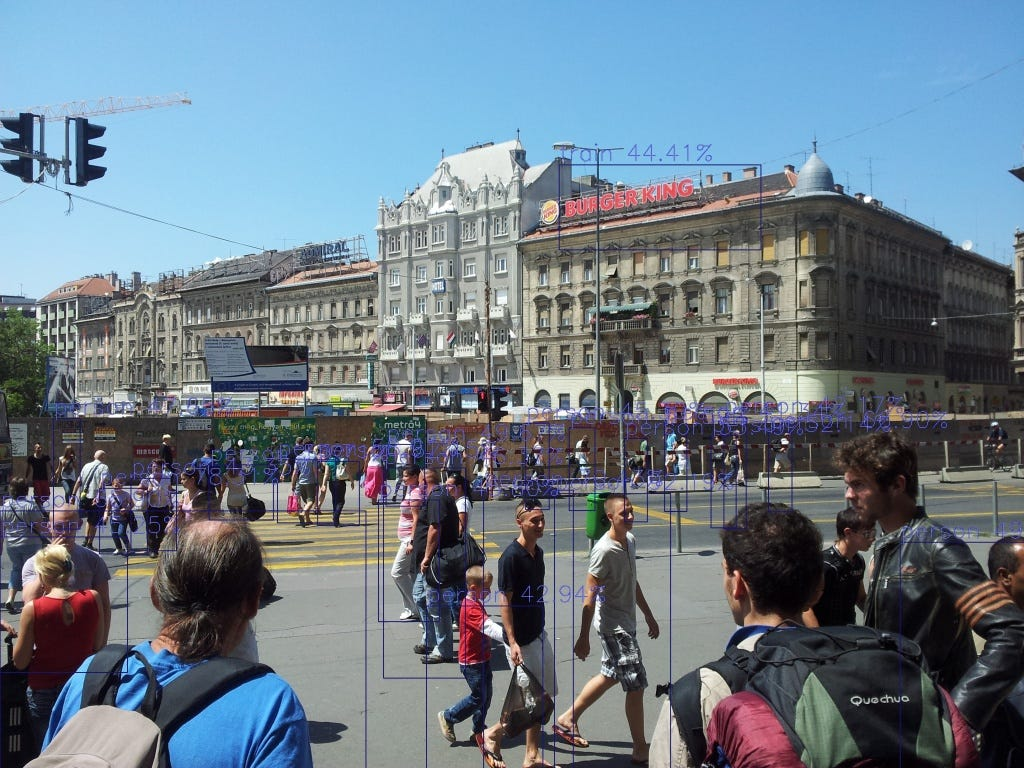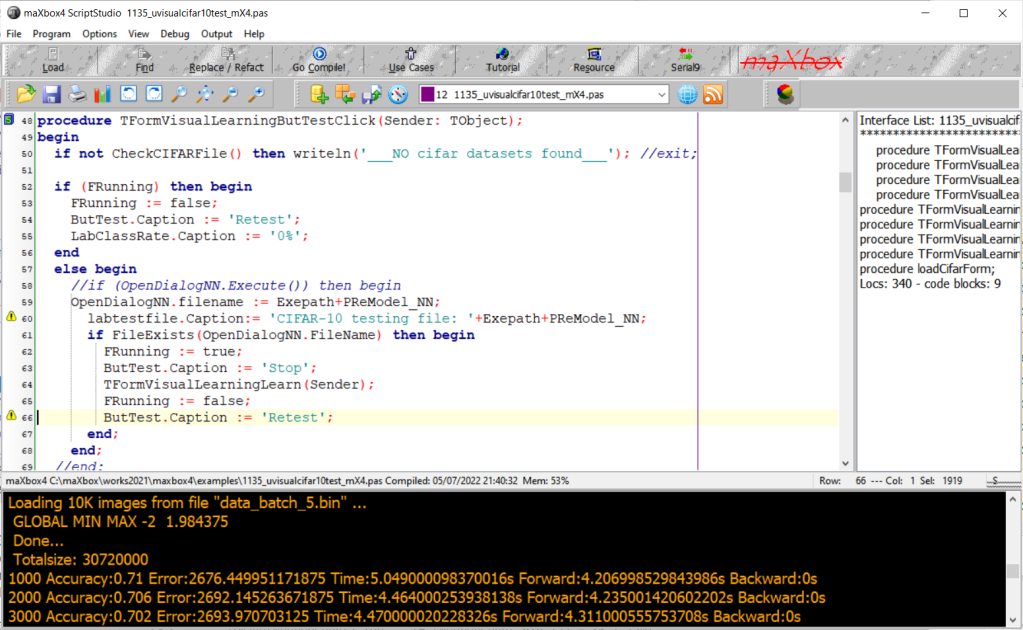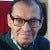# CNN Pipeline Train

This machine learning tutor explains training the so called CIFAR-10 Image Classifier with loading and testing a pre-trained model.

The pre-trained model is: SimpleSeparableImageClassifier124_50_2.nn

This command line tool and script runs the CAI Network with CIFAR10 files; Utility to load cifar-10 image data into training and test data sets based on the script. Download the cifar-10 (python) version dataset from here, and extract the cifar-10-batches-1..5 folder into the same directory as the script (for validating use only test_batch.bin).

CIFAR-10 and CIFAR-100 datasets (toronto.edu)

Note about to build SimpleSeparableImageClassifier

The code contains example usage, and runs under Python3, JupyterNotebook, Lazarus and maXbox4. Note that the load_cifar_10_data() function has the option to load the images as negatives using negatives=True. There are 50000 training images and 10000 test images.

For the first script 1135_Cifar10SeparableConvolution_50.pas you need the 50000 training images (cifar-10-batches-1..5).

For the second script 1135_uvisualcifar10test_mX4_1.pas you need only the 10000 test images.

So we do have the following 10 files as our pipeline:

1. data_batch_1.bin (30,730,000 bytes)
2. data_batch_2.bin (30,730,000 bytes)
3. data_batch_3.bin (30,730,000 bytes)
4. data_batch_4.bin (30,730,000 bytes)
5. data_batch_5.bin (30,730,000 bytes)

1135_Cifar10SeparableConvolution_50.pas to train model (~10 hours)

SimpleSeparableImageClassifier124_50_2.nn output pre-trained model

SimpleSeparableImageClassifier124_50_2.csv result report

6. test_batch.bin (30,730,000 bytes) input to script 1135_uvis..

SimpleSeparableImageClassifier124_50_2.nn input to script

1135_uvisualcifar10test_mX4_1.pas to test and evaluate model

We start with a short introduction to the CIFAR-10 Separable CNN Classification Example SimpleSeparable (comments in code). As I said you don’t need to run the first script (about 9 hours and 2 GByte RAM) because you can experiment and explore direct with the second script and the pre-trained model *.nn and direct test with a TestBatch() procedure.

Adding neurons and neuronal layers is often a possible way to improve artificial neural networks when you have enough hardware and computing time. In the case that you can’t afford time, parameters and hardware, you’ll look for efficiency with Separable Convolutions (SCNN). This is what the first script does:

`NN.DebugWeights(); NN.DebugStructure(); WriteLn('Layers: '+itoa( NN.CountLayers())); WriteLn('Neurons: '+itoa( NN.CountNeurons())); WriteLn('Weights: '+itoa( NN.CountWeights())); CreateCifar10Volumes(ImgTrainingVolumes, ImgValidationVolumes, ImgTestVolumes, csEncodeRGB); NeuralFit:= TNeuralImageFit.Create; NeuralFit.FileNameBase:= 'SimpleSeparableImageClassifier124_50_2'; NeuralFit.InitialLearningRate:= 0.001; //0.001 NeuralFit.LearningRateDecay:= 0.1 //0.01; NeuralFit.StaircaseEpochs:= 10; NeuralFit.Inertia:= 0.9; NeuralFit.L2Decay:= 0.0001; //0.00001; NeuralFit.Fit(NN, ImgTrainingVolumes, ImgValidationVolumes, ImgTestVolumes, {NumClasses=}10, {batchsize=}128, {epochs=}50); NeuralFit.Free;`

As you can see the output is the NeuralFit.FileNameBase. As can be seen on above script, a separable convolution is a composition of 2 building blocks:

• A depth-wise convolution followed by
• a point-wise convolution.

And we can see the progress during learning rate:

• Epochs: 40 Examples seen:1600000 Test Accuracy: 0.7010 Test Error: 0.8364 Test Loss: 0.8692 Total time: 218.73min
• Epochs: 40. Working time: 3.85 hours.
• Epochs: 50 Examples seen:2000000 Test Accuracy: 0.7242 Test Error: 0.7753 Test Loss: 0.8144 Total time: 292.09min
• Epoch time: 3.2000 minutes. 50 epochs: 2.7000 hours.
• Epochs: 50. Working time: 4.87 hours.

Now we jump to the second script as our main topic. The origin is based on a Lazarus Experiment.

Pre-trained models means the models which have been already trained on some sort of data like cifar with different number of classes. Considering this fact, the model should have learned a robust hierarchy of features, which are spatial, rotation, and translation invariant, as we have seen before with regard to features learned by CNN models.

So the pipeline of the process goes like this:

1. Train (or fit) a model to get the feature map with weights.
2. Test the classifier on unseen date with the pre-trained model.
3. Evaluate the classifier with different pre-trained models.

The last point means we can change in our second script the pre-trained model to compare the score or benchmark for better accuracy:

We can compare ImageClassifierSELU_Tutor89_5.nn with SimpleSeparableImageClassifier124_50_2.nn.

`Const PReModel_NN = 'SimpleSeparableImageClassifier124_50_2.nn'; //PReModel_NN = 'SimpleSeparableImageClassifier.nn'; //PReModel_NN = 'SimpleSeparableImageClassifier124.nn'; //PReModel_NN = 'SimpleSeparableImageClassifier124_50_3.nn'; //PReModel_NN = 'ImageClassifierSELU_Tutor89.nn'; NN := TNNet.Create(); writeln('Creating CNeural Network...'); ImgVolumes := TNNetVolumeList.Create(true); NumClasses := 10; fileName:= Exepath+PReModel_NN; //OpenDialogNN.FileName; writeln('Loading neural network from file: '+fileName); NN.LoadFromFile(fileName); NN.EnableDropouts(false); firstNeuronalLayer := NN.GetFirstNeuronalLayerIdx(0); pOutput := TNNetVolume.Create0(NumClasses,1,1,0); //or 1 vOutput := TNNetVolume.Create0(NumClasses,1,1,0); vDisplay:= TNNetVolume.Create0(NumClasses,1,1,0); SetLength(aImage, NN.Layers[firstNeuronalLayer].Neurons.Count); SetLength(sImage, NumClasses);`

Also a view of the neurons is possible the get some insights of the power of segmentation and finally discrimination. So a pre-trained model is a model that was trained on a large benchmark dataset to solve a problem similar to the one that we want to solve. Accordingly, due to the computational cost of training such models, it is common practice to import and use models from published platforms. We use a platform process pipeline ;-).

Lets start with a simple sample to compare (evaluate) this 2 models with our second script:

So it’s obvious that the 2 models are underperformed. There is a list of models and their performance and parameter count here:

https://keras.io/api/applications/

LeNet for example is the most popular CNN architecture it is also the first CNN model which came in the year 1998. LeNet was originally deve-loped to categorise handwritten digits from 0–9 of the MNIST Dataset. It is made up of seven layers, each with its own set of trainable para-meters.

LeNet is a convolutional neural network structure proposed by Yann LeCun et al. in 1989. In general, LeNet refers to LeNet-5 and is a simple convolutional neural network.Convolutional neural networks are a kind of feed-forward neural network whose artificial neurons can respond to a part of the surrounding cells in the coverage range and perform well in large-scale image processing. (Wiki)

`Application.ProcessMessages(); if pOutput.GetClass() = ImgVolumes[ImgIdx].Tag then begin Inc(Hit); //WriteLn(' Tag Label: '+ itoa(ImgVolumes[ImgIdx].Tag)); end else begin Inc(Miss); end;`

To get a better conclusion there’s a simple representation of a neural net with some attributes and a TestBatch() procedure below:

`NN.DebugWeights(); //WriteLn(' Layers: ', NN.CountLayers() ); //WriteLn(' Neurons:', NN.CountNeurons() ); WriteLn('Neural CNN network has: '); WriteLn(' Layers: '+ itoa(NN.CountLayers() )); WriteLn(' Neurons: '+ itoa(NN.CountNeurons() )); WriteLn(' Weights: '+ itoa(NN.CountWeights() )); WriteLn('Computing...'); {Neural CNN network has: Pre-Model: Layers: 13 Layers: 11 Neurons: 205 Neurons: 124 Weights: 20960 Weights: 14432 Computing...} //Directory of C:\maXbox\EKON_BASTA\EKON24\cifar-10-batches-bin\ //This function tests a neural network on the passed ImgVolumes: {procedure TestBatch ( NN: TNNet; ImgVolumes: TNNetVolumeList; SampleSize: integer; out Rate, Loss, ErrorSum: TNeuralFloat ); } rate:= 0; loss:= 0; ErrorSum:= 0; TestBatch(NN, ImgVolumes, 1000, rate, loss, ErrorSum); writeln('Test batch score: '+Format(' Rate:%.4f, Loss:%.4f, ErrorSum:%.4f ', [rate, loss, ErrorSum])); LabClassRate.Caption:= format('Ø %.2f%% ',[rate*100]); end;`

The learning rate is the crucial hyperparameter used during the training of deep convolution neural networks (DCNN) to improve model accuracy; this you can follow in the SimpleSeparableImageClassifier124_50_2.csv.

SimpleSeparableImageClassifier124_50_2.csv.

# Conclusion

The proper way to use a CNN doesn’t exists. The advice for ugly score is to use a smaller learning rate or larger batch size for the weights that are being fine-tuned and a higher one for the randomly initialized weights (e.g. the ones in the softmax classifier) TNNetSoftMax. Pre-trained weights are already good, they need to be fine-tuned, not distorted.

The scripts can be found:

Reference:

Script Ref: 1073__CAI_3_LearnerClassifier22_Tutor_89_2.txt

`Appendix: show neurons from maXbox4 integration*----------------------------------------------------------------------------*) for NeuronCount:= 0 to NN.Layers[firstNeuronalLayer].Neurons.Count- 1 do begin aImage[NeuronCount]:= TImage.Create(FormVisualLearning); aImage[NeuronCount].Parent := FormVisualLearning; aImage[NeuronCount].Width := NN.Layers[firstNeuronalLayer].Neurons[NeuronCount].Weights.SizeX; aImage[NeuronCount].Height := NN.Layers[firstNeuronalLayer].Neurons[NeuronCount].Weights.SizeY; aImage[NeuronCount].Top := (NeuronCount div 12) * 38 + 160; //120 aImage[NeuronCount].Left := (NeuronCount mod 12) * 38 + 60; aImage[NeuronCount].Stretch:=true; end;`CNN Person Detector at Vienna Central Station West, Photo by Silvia RothenThe App in Action

Originally published at http://maxbox4.wordpress.com on July 7, 2022.

--

--## Max Kleiner

Max Kleiner's professional environment is in the areas of OOP, UML and coding - among other things as a trainer, developer and consultant.